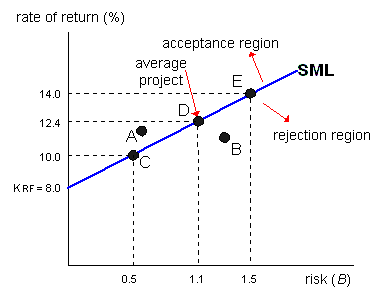### Why should I choose AnalystNotes?

AnalystNotes specializes in helping candidates pass. Period.

### Subject 4. Risk Analysis of Capital Investments - Market Risk Methods

Risk-adjusted discount rates based on market risk measures should be used as the required rate of return for projects when the investors are diversified. The capital asset pricing model (CAPM) and arbitrage pricing theory (APT) are common approaches for finding market-based risk-adjusted rates. We will discuss CAPM below.

In the CAPM, total risk is measured as the standard deviation of project returns. It has two components: systematic risk that cannot be diversified away, and unsystematic risk that can be diversified away. Diversified investors require a risk premium for taking systematic risk only. Therefore, the stand-alone risk measures described in the previous subject are not appropriate when the corporation is diversified. The measure of systematic risk (β) should be used in the CAPM.

Security Market Line (SML) shows how investors are willing to make trade-offs between risk as measured by beta and expected returns. The higher the beta risk, the higher the rate of return needed to compensate investors for bearing this risk. It expresses the following risk/return relationship:

kproject = krisk-free + (kmarket - krisk-free) x Betaproject

The SML can be used to assess a project's market risk.

Assume a firm uses only equity capital. Its cost of equity is also its corporate cost of capital (WACC). If we can determine the beta for each project (Betaproject), then the project cost of capital is kproject = krisk-free + (kmarket - krisk-free) x Betaproject.The SML represents the beta risk of the projects. It indicates the required rate of return for a project, given its risk. The higher the project's beta risk, the higher its required rate of return. If the expected rate of return on a given capital project (project A) lies above the SML, the expected rate of return on the project is more than enough to compensate for its risk, and the project should be accepted. Conversely it should be rejected (project B).

An average-risk project (project D) has the same beta risk as the firm's capital, and thus the project cost of capital equals the corporate cost of capital. If a firm concentrates its new investments in either high- (project E) or low-risk (project C) projects as opposed to average-risk projects, its corporate beta will rise or fall from the current value and its required rate of return on common stock will change from its current value.

#### Practice Question 1

You are analyzing a financial manager's estimate of the cost of equity capital for a project. Which of the following is NOT accurate given the estimate was prepared using the SML approach?

A. The SML applies only to firms with stable dividend growth rates.
B. Like the dividend growth model, the SML generally relies on using the past to predict the future. Unlike the dividend growth model, the SML estimate is adjusted for risk.
C. The quality of the estimate from the SML approach is sensitive to the quality of the estimates of the variables in the model.

#### Practice Question 2

HR Co. has a beta equal to 1.3. It is considering investing in a project that has a beta equal to 1.4. The risk-free rate is equal to 8 percent, and the market expected return is 13 percent. Which of the following statements is false?

A. If we use the beta of the firm in the SML equation we obtain the required return on the stock. If we substitute the project's beta in the SML equation we obtain the project cost of capital.
B. If we substitute the average of the project beta and the firm's beta, i.e., 1.35, we obtain the project's cost of capital.
C. The project cost of capital is 15 percent.

The project's cost of capital is obtained by substituting the project's beta in the SML, in this case it is 15 percent (8 + 1.4 (13 - 8) = 15)

#### Practice Question 3

Which of the following statements is incorrect with regards to the use of a security market line (SML) during the capital budgeting process?

A. Target projects in which expected returns plot below the SML, imply that there are similar risk projects in the market that are yielding a lower return than the targeted project.
B. Projects whose expected returns plot below the SML should be rejected.
C. Projects whose costs are lower than the present value of its cash flows will plot above the SML.